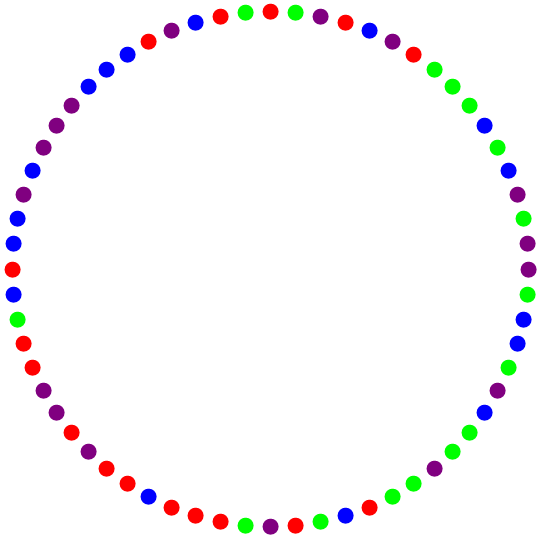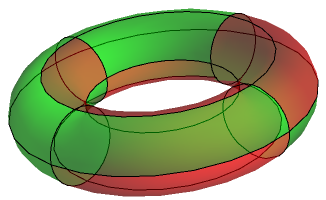# De Bruijn sequences

Given a finite alphabet Σ of size s and an integer n, a de Bruijn sequence is a cyclic string of$s^n$ symbols, such that each n-symbol string over Σ appears exactly once. For example, taking Σ to be an alphabet of four colours and choosing n = 3 gives the following de Bruijn sequence of length 64:It is, quite obviously, the shortest (oriented) loop that contains all possible strings of length 3 over an alphabet of 4 symbols. If we take the alphabet to be the four nucleotide bases of DNA, then this loop of 64 base pairs has the following interesting property: it contains all 64 possible DNA codons.

If one were to actually realise this loop of DNA and effect the process of transcription, then a continual periodic string of RNA would be produced, forming at a site which constantly circumnavigates the DNA loop. Due to the serendipity of 3 and 64 being coprime, this would be translated into a period-64 sequence of all possible amino acids.

Another application of de Bruijn sequences is attempting to break into an electronic safe, which opens when the last four digits pressed matches a particular passcode. The naïve approach of trying each passcode in lexicographical order involves 40000 key presses:

00000001000200030004 [...] 99959996999799989999

Much more efficient is the use of a de Bruijn sequence, which (when the first three digits are re-appended to the end to produce a linear, rather than cyclic, string) is only 10003 digits long.

It can be shown that de Bruijn sequences exist for any ordered pair (s, n), and that the number of such sequences is$\dfrac{(k!)^{k^{n-1}}}{k^n}$. Even more impressive is that the concept generalises to de Bruijn tori, which are known to exist in the square case. For example, here is a$4 \times 4$ torus with 2 colours and every possible$2 \times 2$ block as a subregion:Nathaniel Johnston considers a similar problem, where the shortest linear string containing all permutations of {1, 2, …, n} is desired. Optimal solutions are known for$n \leq 4$, but larger cases are beyond the capabilities of brute-force exhaustive search.

The analogous problem to the electronic safe-cracking is a situation in which a monk has to ring every permutation of six monastery bells. The usual approach is to perform each permutation in succession by use of the Steinhaus-Johnson-Trotter algorithm. However, this particular monk is very lazy, so decides to employ the suspected optimal solution to reduce his workload from 4320 bells to just 873 (see Sloane’s A180632).

This entry was posted in Uncategorized. Bookmark the permalink.

### 0 Responses to De Bruijn sequences

1.Unanimous Cowherd says:

Sadly, the translation of that RNA strand would halt after a short time when one of the three stop codons cropped up.

Is the sequence at the top of the post unique up to rotations, reflections, and permutation of the four colors?

The sequence is, by inspection, asymmetric with respect to the four colors — no two can be exchanged and the whole thing rotated and/or flipped to return it to the original — and by definition has no shorter period (so, no rotational symmetry); the unique occurrences of three-of-a-kind-in-a-rows for each color prohibit reflection symmetry (at most two could lie on the symmetry axis, out of four). Thus, it seems to be devoid of any symmetries.

•apgoucher says:

It is by no means unique. Up to rotation, there are 189321481108517289984 distinct de Bruijn sequences; colour permutations and reflections can only account for 48 of those.